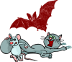# Catz, Ratz, and Batz – Activity Directions

### Catz, Ratz, and Batz Rules

In the Catz, Ratz, and Batz game (which can be purchased online or in game stores) there are nine 6-sided dice. Each die has a 3, a 5, a 7, a cat, a bat, and a rat. To start a turn, each player rolls all the dice. All of the animals are placed to the side. If the remaining numbers match or if there are no numbers, the turn is over. If the numbers do not match, the player chooses to keep all of the dice with matching numbers and rolls the others again. Any animals are set to the side. The player may change the number being kept at any time until only one number remains.

Here are some example scores
• Cat, Cat, Cat, Bat, Rat, 5, 5, 5, 5. Base score: 20. Number of good animals: 3. Number of bad animals: 1. (The bat is neutral so it does not count.) Number of good animals remaining: 2. This means that two copies of the base score will be added to the base score for a total of 60.
• Cat, Cat, Bat, Rat, 3, 3, 3, 3, 3. Base score: 15. Number of good animals: 3. Number of bad animals: 1. Number of good animals remaining: 2. Total score: 45.
• Cat, Bat, Bat, Bat, Rat, Rat, Rat, 3, 3. Base score: 6. Number of good animals: 4. Number of bad animals: 3. Number of good animals remaining: 1. Total score: 12.
• Cat, Cat, Cat, Cat, Bat, 7, 7, 7, 7. Base score: 28. Number of good animals: 4. Number of bad animals: 1. Number of good animals remaining: 3. Total score: 112.

Players take a pre-determined number of turns or play for a certain amount of time and then total their scores.

### Catz, Ratz, and Batz

Play the Catz, Ratz, and Batz game several times so that everyone understands the scoring system. Then consider the questions below.

### What is the Highest Possible Score?

To tackle this question, imagine that you could choose the outcomes of the dice rather than rolling them. You might choose some animals and some numbers. What would be the best animal to choose and what would be the best number to choose?

Make a table showing every possible combination of cats and 7s that could be made with the dice. Make a third column on the table showing the corressponding total scores for each case.

On a coordinate grid, plot the number of cats along the x-axis and the total score along the y-axis. What kind of graph emerges?

Create a formula that gives the total score as a function of the number of cats. Is this function linear, quadratic, polynomial, exponential, rational, or does it have another form? Why do you think the total score function has that form?

### How Much Do Rats Lower Your Potential Score

Suppose that one of the dice shows a Rat. What is the highest score that is possible if you could select the remaining dice rather than rolling them? What if there are two Rats? Three Rats? Four Rats? Five or more Rats?

### What is the Probability That Your Turn Will Only Last One More Roll?

Suppose that you have the following dice in the middle of your turn
Cat, Bat, Bat, Bat, Bat, Rat, 3, 5, 5.

Suppose that you choose to keep the 3. What is the probability that your next roll is your last roll? In other words, what is the probability that re-rolling the two fives will result in either an animal or a 3 on each die?

### What is the Best Bet?

Suppose that you have the following dice in the middle of your turn:

Cat, Cat, Cat, Bat, Bat, Rat, 3, 5, 7.

What are all of the possible score outcomes for your turn if you choose 3s and stick with that choice until the end? What is the probability of each of those outcomes? What is the expected value of the outcome in the case where you choose 3s?

What are all of the possible score outcomes for your turn if you choose 5s and stick with that choice until the end? What is the probability of each of those outcomes? What is the expected value of the outcome in the case where you choose 5s?

What are all of the possible score outcomes for your turn if you choose 7s and stick with that choice until the end? What is the probability of each of those outcomes? What is the expected value of the outcome in the case where you choose 7s?

Which number would be the best bet?

### Another Scenario

Suppose that you have the following dice in the middle of your turn:

Cat, Cat, Cat, Cat, Bat, Bat, 3, 5, 7.

What are all of the possible score outcomes for your turn if you choose 3s and stick with that choice until the end? What is the probability of each of those outcomes? What is the expected value of the outcome in the case where you choose 3s?

What are all of the possible score outcomes for your turn if you choose 5s and stick with that choice until the end? What is the probability of each of those outcomes? What is the expected value of the outcome in the case where you choose 5s?

What are all of the possible score outcomes for your turn if you choose 7s and stick with that choice until the end? What is the probability of each of those outcomes? What is the expected value of the outcome in the case where you choose 7s?

Which number would be the best bet?